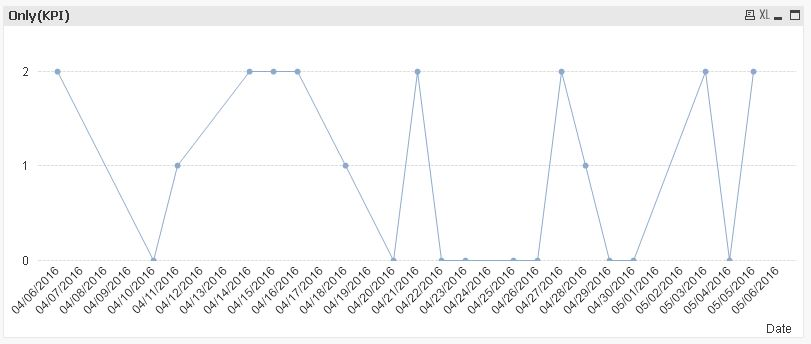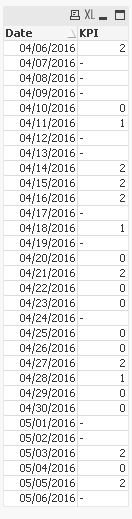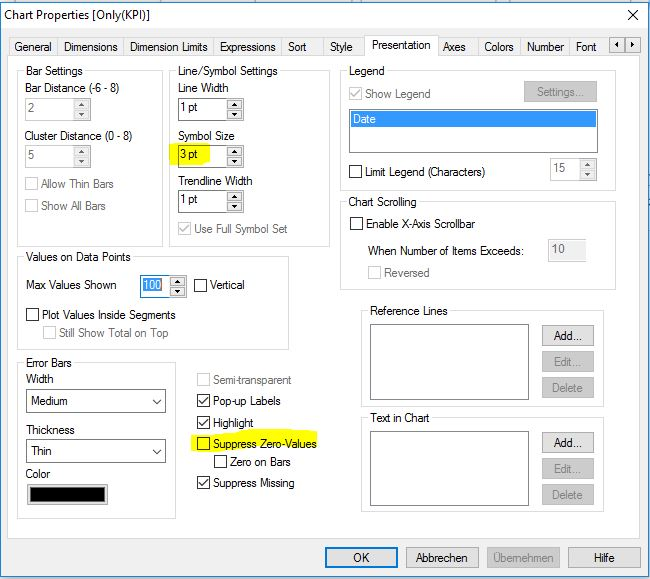# QlikView App Development

Discussion Board for collaboration related to QlikView App Development.

HighlightedNot applicable

## Let QlikView Consider The Difference Between 0-Value and Missing Value

Hi Guys,

Hope you can help me out.

I've got a KPI that shows a number over time. This number is usualy 0 or 1 and sometimes 2. When I want to show this in a Line chart Qlik doesn't show the 0-values, because it thinks these are missing values. I want a solid line in my chart and when the value is 0 it must continu on the x axis. I can't do this with the supress zero-values because I want the check between a 0-value and a missing value.

Tags (5)
1 Solution

Accepted SolutionsNot applicable

## Re: Let QlikView Consider The Difference Between 0-Value and Missing Value

I got it guys. Thanks for the help but I've solved it a different way.

First I upped all the values in the script with 0.0000000001. This way the zero values become larger than zero and the missing values become 0. Then I used the NUM() function to only show the first the value without decimals. This way the value of 0.0000000001 where showed as 0, but are in fact larger than 0. This way I can use the argb() color function to only give a color to the values larger than 0.

4 RepliesMVP

## Re: Let QlikView Consider The Difference Between 0-Value and Missing Value

Maybe something like (assuming a KPI like Sum(Value)):

=If( Sum(Value), Sum(Value), Dual( '0', Pow(2,-27) ) )MVP

## Re: Let QlikView Consider The Difference Between 0-Value and Missing Value

Hi,

maybe marking existing values using symbols helps to distinguish between missing and zero-values when showing zero-values:regards

MarcoNot applicable

## Re: Let QlikView Consider The Difference Between 0-Value and Missing Value

I got it guys. Thanks for the help but I've solved it a different way.

First I upped all the values in the script with 0.0000000001. This way the zero values become larger than zero and the missing values become 0. Then I used the NUM() function to only show the first the value without decimals. This way the value of 0.0000000001 where showed as 0, but are in fact larger than 0. This way I can use the argb() color function to only give a color to the values larger than 0.MVP

## Re: Let QlikView Consider The Difference Between 0-Value and Missing Value

Isn't this pretty similar to what I've suggested above (maybe except using the background color)?

=If( Sum(Value), Sum(Value), Dual( '0', Pow(2,-27) ) )